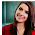# Computer System Architecture Set 28

### Questions 271 to 280

271.
According to the Demorgan’s Theorem, what is the value of F’, where F=P’Q+RS.
(a) (P+Q’)(R’+S’)                                                  (b) (P’+Q’)(R’+S’)        (c) (P+Q’)(R+S)
(d) (P+Q)(R’+S’)                                                   (e) (P’+Q’)(R’+S).
272.
_______________ is a input device, which involves to demonstrate speech recognition.
(a) Speakers                                                          (b) Scanner                    (c) Light pen
(d) Microphone                                                     (e) CD-Player.
273.
Identify the circuit which gives one output from n number of inputs.
(a) Demultiplexer                                                 (b) Multiplexer              (c) Decoder
(d) Encoder                                                            (e) Sequential Circuit.
274.
Subtract the given unsigned decimal numbers by taking the 10's complement of the subtrahend. 6543-4444.
(a) 2098                         (b) 2099                         (c) 2096                         (d) 2097                         (e) 2095.
275.
From the given k-map, the simplified equation for F is __________.
(a) ac+ab’                      (b) a’c+ab                      (c) a’c+ab’                     (d) a’c’+ab’                   (e) a’c+a’b’.
276.
Simplify AB+AB’.
(a) A                               (b) B                               (c) (A’+B’)                     (d) (A+B)                       (e) AB.
277.
The octal number of 011001 is _____________.
(a) 32                              (b) 31                              (c) 34                              (d) 33                              (e) 35.
278.
The 10’s complement of 9999 is ______________.
(a) 9999                         (b) 0000                         (c) 1111                         (d) 0001                         (e) 1000.
279.
Which of the following depicts the figure below
 15 14 13 12 11 0 1 1 1 1

(a) Register reference                                           (b) I/O instruction
(c) Memory reference                                          (d) A and C                                                            (e) A or C.
280.
Identify the formula for XNOR gate.
(a) A’B+B’A                 (b) A’B’+AB                 (c) A+B                          (d) A.B                           (e) A’B.

 271 Answer :   (a) Reason:    According to the Demorgan’s Theorem , the value of F’, where F=P’Q+RS is (P+Q’)(R’+S’). 272 Answer :   (d) Reason:    Microphone is a input device , which involves to demonstrate speech recognition. 273 Answer :   (b) Reason:    The circuit which gives one output from n number of inputs is Multiplexer. 274 Answer :   (b) Reason:    The subtraction of given unsigned decimal numbers by taking the 10's complement of the subtrahend , 6543-4444 is 2099. 275 Answer :   (c) Reason:    From the given k-map, the simplified equation for F is a’c+ab’ 276 Answer :   (a) Reason:    AB + AB’ = A(B + B’) = A. 277 Answer :   (b) Reason:    The octal number of 011001 is 31. 278 Answer :   (d) Reason:    The 10’s complement of 9999 is 0001. 279 Answer :   (b) Reason:    The figure depicts Input output instruction as the last four bits are 1’s. 280 Answer :   (b) Reason:    The formula for XNOR gate is A’B’+AB.

29  30  31  32  33  34  35  36  37  38  39  Next >>

#### 1 comment :

1.It has been simply incredibly generous with you to provide openly what exactly many individuals would’ve marketed for an eBook to end up making some cash for their end, primarily given that you could have tried it in the event you wanted.

AWS Training in Bangalore

Python Training in Bangalore